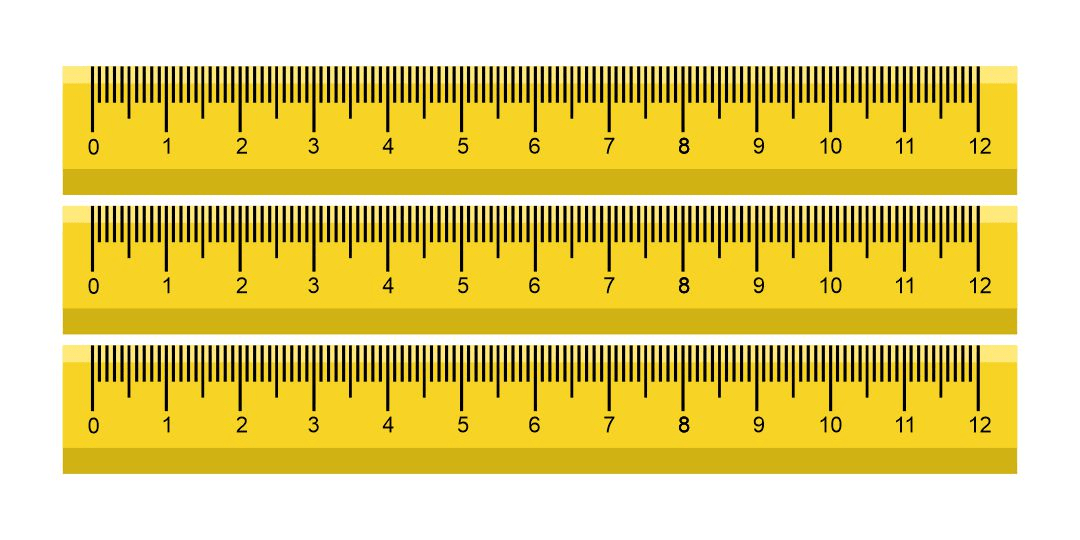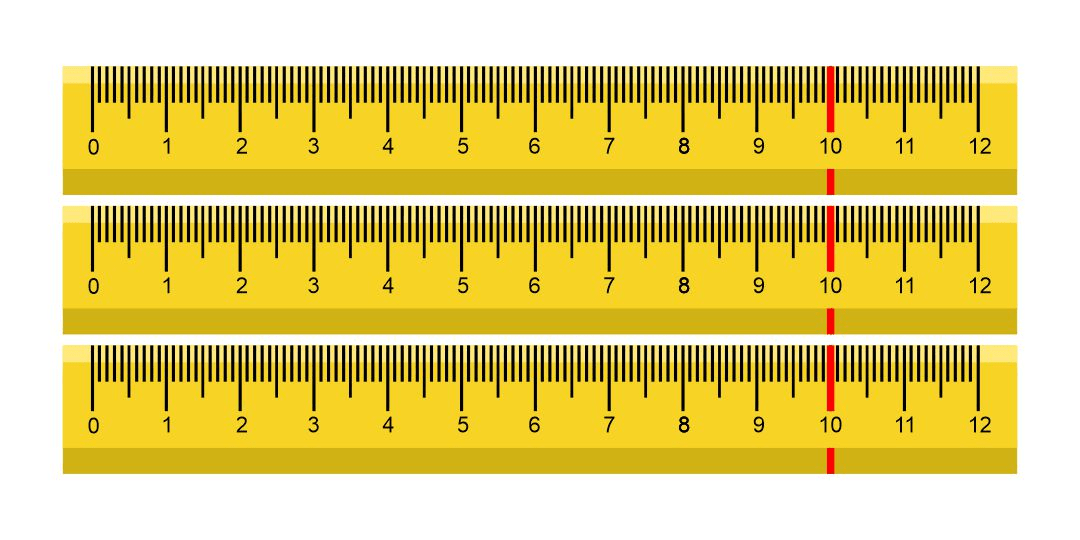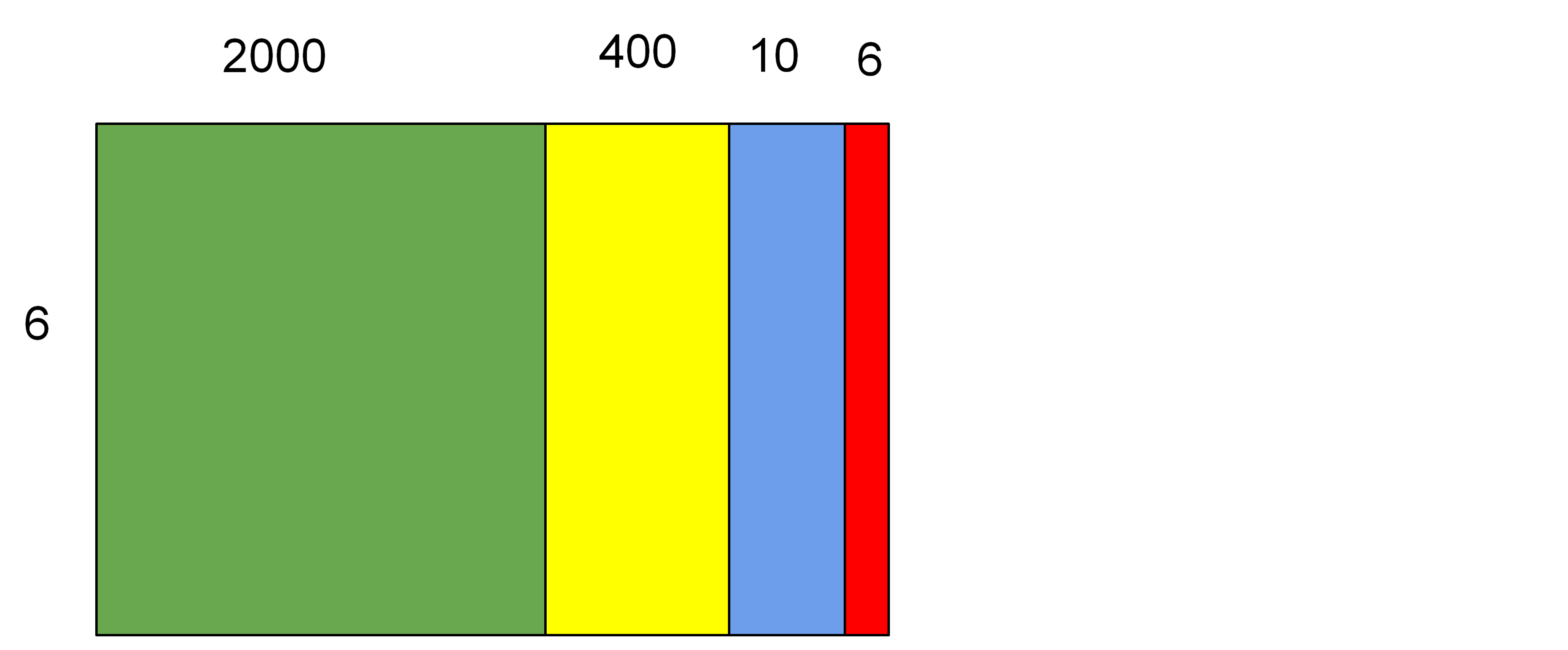Listen to this Lesson:## Multiplying 3 and 4 digit Numbers using Area Models

Multiplying multi-digit numbers using area not only helps students to visualize the products, but also helps show them how partial products work and add up.  This can help students learn and understand multiplication as well as apply it to the standard algorithm for multiplication. In this activity we will be Multiplying 3 and 4 digit Numbers using Area Models.

### Area and Multiplication

Area is a great way to show how multiplication works.  Students learn arrays and see groups of numbers.  Being able to see the area is just like making rows or groups of numbers.  It can also be used for real life applications.

We have tiles on the floors of the hallways at school.  I like to bring my class up to the hallway and give them an area to calculate.  We step on the tiles and see how the squares make up the area.  One way to find the area is to count each tile.  But students soon start to see equal rows of tiles and they figure out ways to use multiplication too!

### Area Puzzles

I also like to use Area puzzles with my students.  It’s a great way to use multiplication and find the missing sides and dimensions.  Area is a geometry skill but it is also a visual way to look at multiplication.  Area models can also be used when students start learning algebra and factoring equations.  So if fourth graders can learn ways to see multiplication with area, they can build an understanding that will help them when they use the distributive property and when they explore factors and multiples.

AREA is an ARRAY of squares.  So find some tiles on the floor and grab some graph paper – it is time to teach multiplication by using AREA and place value–and students even get to color!

## Set up for the Multiplying 3 and 4 digit Numbers using Area Models Activity

Materials needed:

### Launch the Multiplying Multi-digit Numbers using Area Models Activity

Grab 3 rulers and hold one up.  Ask the class how many inches long it is. Show how there are 12 inches.  Then hold up 2 rulers, and ask how many inches.  They should say 24. Stack the rulers to show that it is 2 rulers of 12 inches each.  Then hold a third ruler up in the stack.  Like the image below:Tell the students that you have 3 rows of 12 inches.  And show that it is equal to 36.

3 x 12 = 36

Then ask how students know the answer.  Some may count 12 + 12+ 12

Others may know what 3 x 12 is.

Then ask if there’s a way to split 12 into place value.  10 + 2

Place a piece of tape at the 10 inch mark on each ruler.

Line them up again.Explain how multiplication can be made easier if we split larger numbers into place value.

So we can think of this as 3×10 = 30 plus 3 rows of 2, 3×2= 6

30 + 6 = 36

Then show the students how to draw area models on graph paper.

Tell them to try and show a rectangle that is 27 by 5.

5 rows of 27 – as a rectangle.

Then have the students use a blue marker or colored pencil and place dots along the top row counting out 20 – shade it in.  Shade in the same 20 in each row.

Ask students to figure out the area of the shaded region (5 rows of 20).

Then have them shade the other part red, and calculate the area (5 rows of 7).

5×20 = 100 (blue)

5 x 7 = 35 (red)

Answer = 100 + 35 = 135

Then ask  “Do you think we can use these models to figure out how to multiply 3 digit numbers? “

“Let’s come up with a color code!”

“If we use BLUE for the 20, that’s in the tens place

We used RED for the ones place

Let’s use YELLOW for the hundreds place.”

At this point, you will have to give students several pieces of graph paper and help them tape them end to end.

“If we have 3 rows of 124, then we would have to count 100 squares!  That’s a lot.  We can model it, but then I can show you an easier way to use the concept and not use too much paper”

Help students draw, shade and model 124 x 3

Similar to the image below:Then explain that we can show the same concept by drawing the rectangle and labeling the sections by place value – even if we don’t have 100 boxes to count, we can label it as 100.

Ask why or when we may need to just label the sides, versus counting them all out.

“124 was hard to count, what if we wanted to do a 4 digit number like 1328 x 7?”

Students may start to realize that we can’t always count the squares, but with multiplication and area, we can show the area and the dimensions.

Use graph paper to show there are only 44 squares across one sheet of paper.  So in order to do larger numbers, the students will need paper taped together.  Otherwise, as the numbers get bigger, students can still use the graph paper to sketch a rectangle, but they do not need to count the boxes.  Instead, they can just split the length into place value, label and color code it.

The width should be the single digit number.  This is one dimension.  The length is the 3 or 4 digit number.  Show the whole length and then color code to show it split by place value.  Here is an example:The dimensions are the width and length.

Explain that when the numbers are larger, they can’t count out 1000 squares on graph paper, but if they draw the area model, they can label each color with the place value numbers.

To show place value, the larger number is turned into expanded form along the length.

Once students see how to split the number, they can color code each rectangle.

Then to find the area of the whole rectangle, they find the area of each colored box.

Review how to multiply by a power of 10.   For example:

3 x 2 = 6

3 x 20 = 60

3 x 200 = 600

3 x 2000 = 6000

Now tell the students that they will work in groups of 4.

Each student gets to make up a multiplication problem and then model it on a piece of graph paper.  But DO NOT SHOW each other yet!  They will write the multiplication problem on the index card and model or count the boxes and shade with our color code on the graph paper.  Then as a group, they will mix up their cards and models, then trade with another group.  Each group works to find the match of the multiplication problem and its area model.

There will be at least 4 rounds.

Round 1:  2 digit by 1 digit – with graph paper and counting the squares

Round 2: 3 digit by 1 digit – less than 130 x 1 digit (graph and count squares)

Round 3: 3 digit by 1 digit – Modeled, with dimensions labeled (not all squares need to be counted)

Round 4: 4 digit by 1 digit – Modeled, with dimensions labeled

(Round 5 : 4 digit by 1 digit – Modeled, with dimensions labeled)

Remind the student to remember to use the color code and count or label carefully.

## Matching Activity

### Make Area Models for multiplying 3 and 4 digit numbers by 1 digit

• Split the students into groups of 4 or 5.
• Each student will create a multiplication problem and write it on an index card.
• Then they will model that problem on graph paper.
• Follow the instructions for each round:

Round 1:  2 digit by 1 digit – with graph paper and counting the squares

Round 2: 3 digit by 1 digit – less than 130 x 1 digit (graph and count squares)

Round 3: 3 digit by 1 digit – Modeled, with dimensions labeled (not all squares need to be counted)

Round 4: 4 digit by 1 digit – Modeled, with dimensions labeled

Round 5 : 4 digit by 1 digit – Modeled, with dimensions labeled

• Once all 4 students have made their problems for Round 1, and modeled it with an area model, have them check each other’s work to make sure they match the problem on the index card.
• Tell the groups to mix up the models and cards and trade their work with another group.
• Now each group tries to match the new problems and models.
• On the back of the index card, write and solve the dimensions of each colored rectangle, then add it up to find the area of the whole model.
• When they think they are finished, the original group can check the work.
• Move to Round 2 – create new problems and matching area models.
• Check work and trade with another group.
• Match problems to correct area models (check with the original group).
• Play continues for each round.
• Note – remember that Round 3,4,5 will involve sketched models – there are not enough grids on graph paper to make rows longer than 140.

Be sure to walk around and help students with their area models.

It’s ok if the larger number models have labels that are not to scale.  Just make sure that students label and split the 3 and 4 digit numbers by place value.

Here’s an example of

2,416 x 6When the students find the match, they must write the area of each color region on the back of the card. Then show what the total area of the rectangle is the sum of these parts.  The total area is the solution to the multiplication problem on the front of the card. It should look like this to prove what the answer is.

6 x 2000 =     12000   GREEN

6 x 400    =     2400   YELLOW

6 x 10       =     60   BLUE

6×6           =     36   RED

### Reflecting on the activity

Walk around and observe how the students work together and help check each other’s work.

• How does the area and splitting by place value make larger numbers easier to multiply?
• Which problems were harder? Which were easier?
• How does the color coding help?
• What would we need to do to show the 4 digit multiplication with the correct number of squares?
• How did you decide to model and label when you couldn’t count squares?

## Extensions

• Mash-up Math has area puzzles to practice Area and multiplication. Here is an example puzzle.
• Show area multiplication with lego bricks.
• Students can create a video or lesson or presentation of how area puzzles work. Here is a Video – explanation on Khan Academy.

## FREE Multiplying 3 and 4 digit Numbers using Area Models Worksheets and Resources

These are all PDF Files. They will open and print easily. The Student Edition Files are labeled SE and the Teacher Editions Files are labeled TE. Click the links below to download the different resources.

## Multiplying 3 and 4 digit Numbers using Area Models Worksheets and Resources

To get the Editable versions of these files Join us inside the Math Teacher Coach Community! This is where we keep our full curriculum of 4th Grade Math Lessons and Activities.